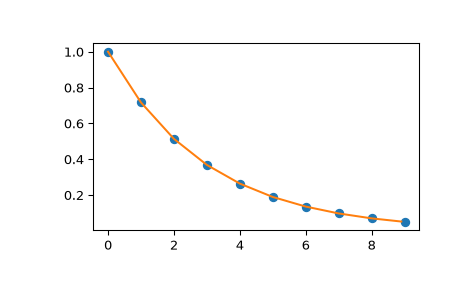# scipy.interpolate.interp1d¶

class scipy.interpolate.interp1d(x, y, kind='linear', axis=-1, copy=True, bounds_error=None, fill_value=nan, assume_sorted=False)[source]

Interpolate a 1-D function.

x and y are arrays of values used to approximate some function f: y = f(x). This class returns a function whose call method uses interpolation to find the value of new points.

Note that calling interp1d with NaNs present in input values results in undefined behaviour.

Parameters
x(N,) array_like

A 1-D array of real values.

y(…,N,…) array_like

A N-D array of real values. The length of y along the interpolation axis must be equal to the length of x.

kindstr or int, optional

Specifies the kind of interpolation as a string (‘linear’, ‘nearest’, ‘zero’, ‘slinear’, ‘quadratic’, ‘cubic’, ‘previous’, ‘next’, where ‘zero’, ‘slinear’, ‘quadratic’ and ‘cubic’ refer to a spline interpolation of zeroth, first, second or third order; ‘previous’ and ‘next’ simply return the previous or next value of the point) or as an integer specifying the order of the spline interpolator to use. Default is ‘linear’.

axisint, optional

Specifies the axis of y along which to interpolate. Interpolation defaults to the last axis of y.

copybool, optional

If True, the class makes internal copies of x and y. If False, references to x and y are used. The default is to copy.

bounds_errorbool, optional

If True, a ValueError is raised any time interpolation is attempted on a value outside of the range of x (where extrapolation is necessary). If False, out of bounds values are assigned fill_value. By default, an error is raised unless fill_value="extrapolate".

fill_valuearray-like or (array-like, array_like) or “extrapolate”, optional
• if a ndarray (or float), this value will be used to fill in for requested points outside of the data range. If not provided, then the default is NaN. The array-like must broadcast properly to the dimensions of the non-interpolation axes.

• If a two-element tuple, then the first element is used as a fill value for x_new < x and the second element is used for x_new > x[-1]. Anything that is not a 2-element tuple (e.g., list or ndarray, regardless of shape) is taken to be a single array-like argument meant to be used for both bounds as below, above = fill_value, fill_value.

New in version 0.17.0.

• If “extrapolate”, then points outside the data range will be extrapolated.

New in version 0.17.0.

assume_sortedbool, optional

If False, values of x can be in any order and they are sorted first. If True, x has to be an array of monotonically increasing values.

splrep, splev

Spline interpolation/smoothing based on FITPACK.

UnivariateSpline

An object-oriented wrapper of the FITPACK routines.

interp2d

2-D interpolation

Examples

>>> import matplotlib.pyplot as plt
>>> from scipy import interpolate
>>> x = np.arange(0, 10)
>>> y = np.exp(-x/3.0)
>>> f = interpolate.interp1d(x, y)

>>> xnew = np.arange(0, 9, 0.1)
>>> ynew = f(xnew)   # use interpolation function returned by interp1d
>>> plt.plot(x, y, 'o', xnew, ynew, '-')
>>> plt.show()Attributes
fill_value

The fill value.

Methods

 __call__(self, x) Evaluate the interpolant

#### Previous topic

Interpolation (scipy.interpolate)

#### Next topic

scipy.interpolate.interp1d.__call__